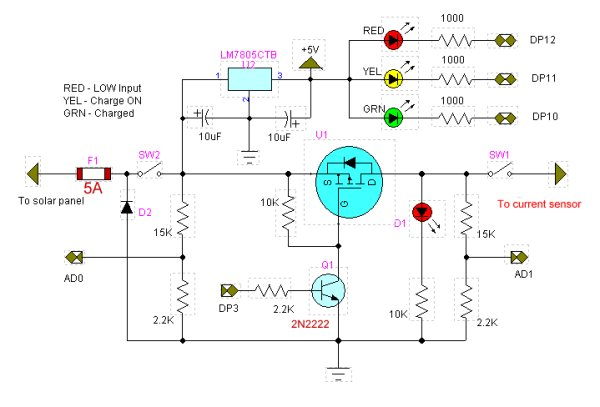# Solar Panel Battery Charge Controller Using Arduino

This is an updated version of Solar Panel Charge Controller Using Arduino.

Solar Panel Battery Charge Controller Using Arduino

Pictures of Power box and Arduino solar charge regulator:
Charge Regulator with Power Box
Connections to Regulator
Inside the Box

The output voltage of the ACS712 is 2.5V with no input while the spec sheet specifies 66 to 185 mV/A output sensitivity. That’s a broad range and given the errors of Arduino’s 10-bit ADC this gives approximate current output. Good enough for most applications.

The LED1 indicator ‘bad’ meaning the input voltage below the charging voltage when on.DP3 turns on charge switch MOSFET transistor. Can use PWM or a simple timing routine. Will blink on/off with charge cycle. (Charge enable). The LED in the new circuit is merely an indicator.

LED2 indicator fully charged battery. (DP10)

A 10-bit analog-to-digital converter (ADC) has a step voltage of about 4.9 mV over a 5-volt range. This relates to the charge point (CP) variable.

To measure input voltage from the solar panel and the voltage on the battery we use a voltage divider to drop the voltage below 5-volts.

This uses two resistor voltage dividers (15k and 2.2k) which produces a voltage of about 1.7 – 1.9 volts when fully charged. This equates to about decimal 346 – 388 (when divided by 4.9mV) from the ADC and is compared to the charge point variable CP.

```
/* Solar cell battery charger/regulator

Refer to:

http://www.bristolwatch.com/solar_charger.htm

DP12 LED1 indicator 'bad' meaning the input
voltage below charging voltage.

DP11 indicates charge on.

DP10 LED2 indicator fully charged battery.

DP3 turns MOSFET ON-OFF and can be
used for PWM.

AD2 measure current from ACS712 Hall
sensor for current measurement.

This uses two resistor voltage dividers
(15k and 2.2k) which produces a voltage
of about 1.7 - 1.9 volts when fully charged.
This equates to about decimal 346 - 388
(when divided by 4.9mV) from the ADC and
is compared to the charge point variable CP.

Note the 4.9 mV was derived from the
10-bit ADC which equals 1023: 5V / 1023 = 4.9mV

Note line "chon = CP - y * 100" when
uncommented the charge 'on' time will
decrease gradually as battery is more
charged. When fully charged the charge voltage
is disabled.

This also has the ability to monitor system
settings through the serial port.

*/

#define LED1 12 // red low Vin
#define LED2 10 // green charged
#define  ChargeON 11 // charge current on
#define POWenable 3

// for use with PWM DP3
#define FULL 255
#define HALF 128
#define QUARTER 64
#define OFF 0

int x;
int y;

// these two variables can be changes
// for differing on/off times.
int chon = 5000; // charge on time
int choff = 2000; // charge off time

int CP = 310; // charge point variable
// CP sets the battery charge voltage level. Can vary from 300-340.
void setup()   {

pinMode(LED1, OUTPUT);
pinMode(LED2, OUTPUT);
pinMode(ChargeON, OUTPUT);
pinMode(POWenable, OUTPUT);

digitalWrite(LED1, HIGH);
digitalWrite(LED2, HIGH);
digitalWrite(ChargeON, HIGH);
digitalWrite(POWenable, LOW);

Serial.begin(9600);

delay(500);}

void loop()   {

Serial.print("CP = ");
Serial.println(CP);

// voltage from solar panel
//under CP on ADC indicates not
// enough voltage to charge battery
// ~ 0.041 is derived from reading the
// voltage with a DVM
// the dividing by the returned
// int value.
Serial.print(x);
Serial.print("   ");
Serial.print("Vin = ");
Serial.println(x * .041);

// voltage on battery to be charged
// over or equal to CP on ADC indicates
// fully charged battery
Serial.print(y);
Serial.print("   ");
Serial.print("Vbat = ");
Serial.println(y * .041);

// red LED
if (x < CP) digitalWrite(LED1, LOW);
else digitalWrite(LED1, HIGH);
// LED on indicates low or no input voltage

// green LED
if (y > CP) digitalWrite(LED2, LOW);
else if (y <= CP) digitalWrite(LED2, HIGH);
// LED on means battery is chargedif ((x > y) && (y <= CP) && (x > CP))  {
// check input voltage and voltage on battery
// turn on charge cycle if voltage input
// good AND battery voltage low.

if (y <= CP)   { // turn on voltage to battery
// uncomment below will shorton ON time as charge
// nears completion.
// chon = (CP - y) * 100;

// turn on charge LED
digitalWrite(ChargeON, LOW);

// charge switching transistor
// can be pulse-width modulated
// four values are OFF, QUARTER, HALF, FULL
// This uses DP3.
analogWrite(POWenable, FULL);

// print chon value
Serial.print("chon = ");
Serial.print(chon);
Serial.print("   ");

delay(2000);

// this section read a Hall effect current sensor
// 1 amp causes a change of 40 or .2v as measured
// with a DVM. Thus .2 / 40 = .025
// This can be used to display charge current.
// float temp = (analogRead(Ioutput) - 510) * .025;
// This could tested for heavy charge current from
// a nearly dead battery which can be limited to
// a safe value by cutting the duty cycle back
// POWenable to QUARTER or HALF.
Serial.print(temp);
Serial.print("    I out = ");
Serial.print((temp - 506) * .0283);
Serial.println(" amps. ");

delay(chon); // ON wait
digitalWrite(ChargeON, HIGH); // Charge LED off
analogWrite(POWenable, OFF);  // turn off MOSFET
}

} // end if

// turn off charge enable
delay(choff); // OFF wait
}
```

For more detail: Solar Panel Battery Charge Controller Using Arduino